• 基于MATLAB对数据库进行的贝叶斯分类器设计
• 贝叶斯详解 ...这里再说贝叶斯分类器设计步骤： 1.对每个簇的数据求均值mu 和协方差矩阵sigma 2.对测试数据，将其对每个簇用均值和协方差矩阵求相关性。 3.将数据分类到相关性大的簇中。 matlab
贝叶斯详解

网上有很多文章介绍贝叶斯原理，这里推荐个链接。 http://blog.csdn.net/anneqiqi/article/details/59666980

贝叶斯分类器

这里再说贝叶斯分类器的设计步骤：
1.对每个簇的数据求均值mu 和协方差矩阵sigma
2.对测试数据，将其对每个簇用均值和协方差矩阵求相关性。
3.将数据分类到相关性大的簇中。

matlab代码

分类器函数 bayesClassifer.m

function [ labels ] = bayesClassifer(trainData,trainLabel,K,testData)
%trainData 训练数据
%trainLabel 训练数据的标签
%K 训练数据的类数
%testData 测试数据
[~,dimentr]=size(trainData);
[numsts,dimente]=size(testData);
if dimentr~=dimente
disp('错误！测试数据和训练数据维数不一样！');
return;
end
mu=zeros(K,dimentr);%各个类的均值
sigma=zeros(dimentr*K,dimentr);%各个类的协方差
%计算各个类的均值和协方差
for i=1:K
mu(i,:)=mean(trainData(trainLabel==i,:));
sigma(((i-1)*dimentr+1):i*dimentr,:)=cov(trainData(trainLabel==i,:));
end
%对测试数据对各个类进行比对
labels=zeros(numsts,1);
for i=1:numsts
P=zeros(1,K);
for j=1:K
%求相关性函数
P(j)=mvnpdf(testData(i,:),mu(j,:),sigma((j-1)*dimentr+1:j*dimentr,:));
end
[~,maxIndex]=max(P);
labels(i)=maxIndex;
end
%可视化
color = {'r.', 'g.', 'm.', 'b.', 'k.', 'y.'};
if dimentr==2
subplot(1,2,1);
for i=1:K
plot(trainData(trainLabel==i,1),trainData(trainLabel==i,2),char(color(i)));
hold on;
end
title('训练数据');
subplot(1,2,2);
for i=1:K
plot(testData(labels==i,1),testData(labels==i,2),char(color(i)));
hold on;
end
title('测试数据');
elseif dimentr==3
subplot(1,2,1);
for i=1:K
plot(trainData(trainLabel==i,1),trainData(trainLabel==i,2),trainData(trainLabel==i,3),char(color(i)));
hold on;
end
title('训练数据');
subplot(1,2,2);
for i=1:K
plot(testData(labels==i,1),testData(labels==i,2),trainData(trainLabel==i,3),char(color(i)));
hold on;
end
title('测试数据');
else
disp('维度不符合画图标准（1维的懒得画），咱就不画啦');
end

end


测试数据生成和分类。

clear;
clc;
n=100;
%生成训练数据
mu1=[2 10];
mu2=[10 2];
sigma1=[1.5 0;0 1];
sigma2=[1 0.5;0.5 2];
r1=mvnrnd(mu1,sigma1,n);
r2=mvnrnd(mu2,sigma2,n);
trainData=[r1;r2];
trainLabel=[ones(100,1);2*ones(100,1)];
%生成测试数据
mu1=[5 5];
n=200;
sigma1=[0.5 0;0 0.5];
testData=mvnrnd(mu1,sigma1,n);
%测试
labels=bayesClassifer(trainData,trainLabel,2,testData);

实验结果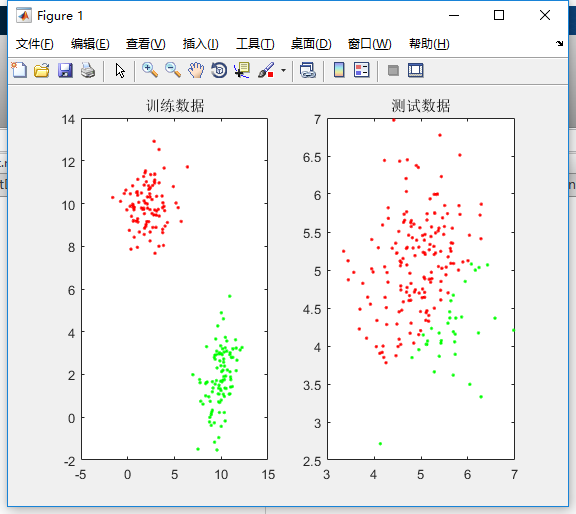展开全文• matlab贝叶斯分类器 身高体重作特征的Bayes分类器设计
• 对数据集的分类，设计参数估计下的最小误判分类器和最小风险分类器，再考虑非参数估计下的贝叶斯分类，比较分析。</p>深度学习 测试用例
• 本文在MATLAB平台上对最小错误率贝叶斯决策和最小风险贝叶斯决策进行测试，比较和分析了实验结果。
• Wine数据集分类——贝叶斯分类算法（MATLAB实现）一、Wine数据集的介绍1.1 实验目的1.2 数据介绍1.3 数据来源二、贝叶斯算法理论2.1 贝叶斯分类2.2 贝叶斯公式2.3 朴素贝叶斯分类器设计流程2.3 朴素贝叶斯分类器的...
Wine数据集分类——贝叶斯分类算法（MATLAB实现）一、Wine数据集的介绍1.1 实验目的1.2 数据介绍1.3 数据来源二、贝叶斯算法理论2.1 贝叶斯分类2.2 贝叶斯公式2.3 朴素贝叶斯分类器的设计流程2.3 朴素贝叶斯分类器的数学原理三、MATLAB仿真实现
一、Wine数据集的介绍
1.1 实验目的

该实验的Wine数据集来自于UCI机器学习数据库中，数据集中的数据则是产自意大利同一地区但来自三个不同品种的葡萄酒进行化学分析的结果，分析确定了三种酒中每种所含13种不同成分的数量。
实验的目的就是利用分类算法实现Wine数据集中三种不同品种的葡萄酒分类。通过学习贝叶斯分类相关的知识，我们决定使用贝叶斯分类算法对Wine数据集进行分类。Wine数据集含有178个样本，我们将采用k折交叉验证的方法选取训练集和测试集，并采用朴素贝叶斯分类算法实现Wine数据集的分类。实验过程所使用的编程软件为MATLAB仿真软件，利用MATLAB实现算法及分类。

1.2 数据介绍
下表是Wine数据集的相关信息。

Data Set Characteristics:
Multivariate
Number of Instances:
178
Area:
Physical

Attribute Characteristics:
Integer, Real
Number of Attributes:
13
Date Donated:
1991-07-01

Associated Tasks:
Classification
Missing Values?
No
Number of Web Hits:
1509517

这些数据包括了三种酒中13中不同成分的数量。13种成分分别是：Alcohol、Malic acid、Ash、 Alkalinity of ash、Magnesium、Total phenols、Flavanoids 、Nonflavanoid phenols、Proanthocyanins、Color intensity、Hue、OD280/OD315 of diluted wines、Proline。在‘Wine.data’文件中，每一行代表一种酒的样本，共有178个样本；一共14列，其中第一列为类别标志属性，共有3类，分别标记为‘1’、‘2’、‘3’，对于三种不同的葡萄酒；后面13列为每一个样本对应属性的属性值；类别‘1’共有59个样本，类别‘2’共有71个样本，类别‘3’共有48个样本。
由于数据集中每个样本的数据都是完整的，没有空缺值，所以没有对该数据集进行必要的数据清洗工作。

1.3 数据来源
http://archive.ics.uci.edu/ml/datasets/Wine
二、贝叶斯算法理论
2.1 贝叶斯分类

贝叶斯分类是指通过训练集（已分类的数据子集）训练来归纳出分类器，并利用分类器对未分类的数据（可以看作是测试集）进行分类。其基本思想是依据先验概率、类条件概率的信息，并按照某种准则使分类结果从统计上讲是最佳的。它是一种有监督学习的分类方法。

2.2 贝叶斯公式

若已知总共有M类样品，以及各类在n维特征空间的统计分布，根据概率知识可以通过样品库得知各类别$w_i(i=1,2,...,M)$的先验概率$p(w_i)$以及类条件概率$p(X/w_i)$。对于待测样品，贝叶斯公式可以计算出该样品分属各类别的概率，叫做后验概率$p(w_i/X)$ 。比较各个后验概率，把X归于后验概率最大的那一个类。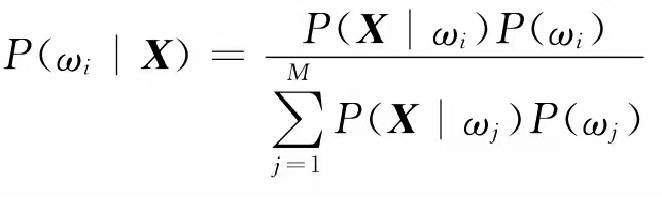2.3 朴素贝叶斯分类器的设计流程

首先，朴素贝叶斯分类其实就是基于最小错误率的贝叶斯分类，同时也称最大后验概率的贝叶斯分类。朴素贝叶斯分类的整个流程如下图所示：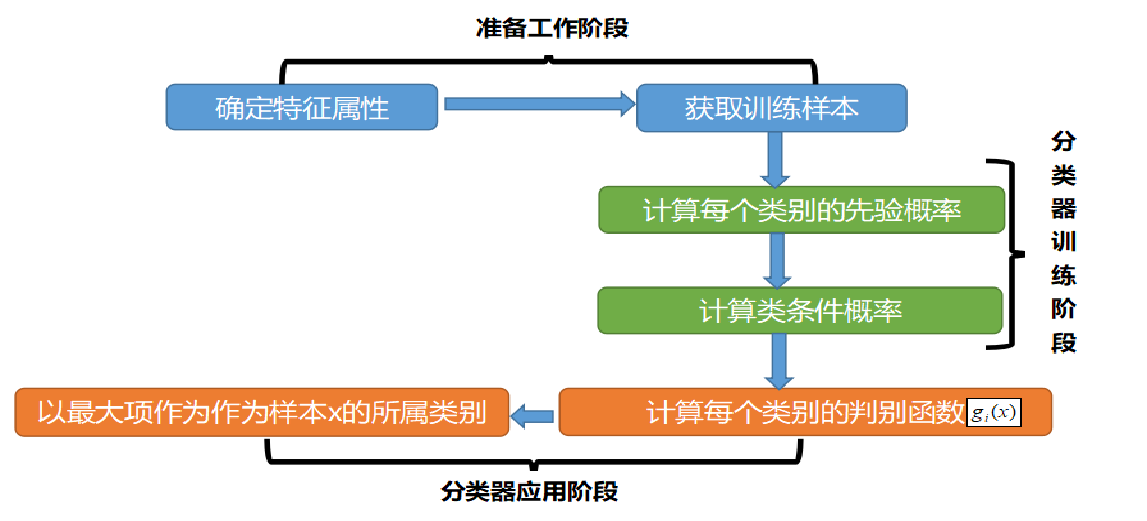可以看到，整个朴素贝叶斯分类分为三个阶段：
第一阶段——准备工作阶段，这个阶段的任务是为朴素贝叶斯分类做必要的准备，主要工作是根据具体情况确定特征属性，并对每个特征属性进行适当划分，然后由人工对一部分待分类项进行分类，形成训练样本集合。这一阶段的输入是所有待分类数据，输出是特征属性和训练样本。这一阶段是整个朴素贝叶斯分类中唯一需要人工完成的阶段，其质量对整个过程将有重要影响，分类器的质量很大程度上由特征属性、特征属性划分及训练样本质量决定。
第二阶段——分类器训练阶段，这个阶段的任务就是生成分类器，主要工作是计算每个类别在训练样本中的出现频率及每个特征属性划分对每个类别的条件概率估计，并将结果记录。其输入是特征属性和训练样本，输出是分类器。这一阶段是机械性阶段，根据前面讨论的公式可以由程序自动计算完成。
第三阶段——应用阶段。这个阶段的任务是使用分类器对待分类项进行分类，其输入是分类器和待分类项，输出是待分类项与类别的映射关系。这一阶段也是机械性阶段，由程序完成。

2.3 朴素贝叶斯分类器的数学原理

本实验中所采用的贝叶斯分类算法是朴素贝叶斯分类算法。朴素贝叶斯算法又叫做基于最小错误率的贝叶斯分类算法，也可称为基于最大后验概率的贝叶斯分类算法。它的原理就是利用贝叶斯公式将样本划分到后验概率最大的那一类中。因此可以定义每一类的判别函数为：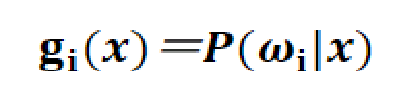由于在同一个模式识别问题中，对于不同的类，贝叶斯公式中的全概率是相同的，所以，各类的判别函数也可以定义为：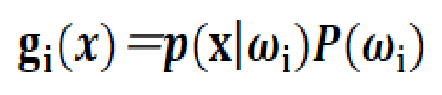假设样本空间被划分成c个类别决策区域，则分类判决规则为：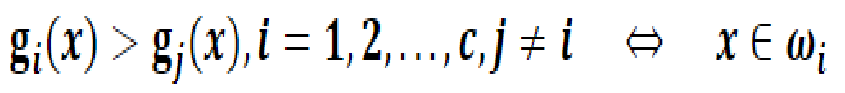此时，任意两个类别之间的决策边界由下述方程决定: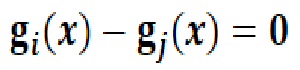先分析一下判别函数。在判别函数中，先验概率是一个与特征向量无关的常量，类条件概率密度则满足一定的概率分布。因为类条件概率与我们要识别的样本x有关。可以说，类条件概率是x的函数。对于一个类别的所有样本，x的取值会呈现出某种概率分布，如果我们知道这个概率分布，也就知道了类条件概率密度，那么贝叶斯分类的分类决策规则也就确定下来了。类条件概率具有不同的概率分布，相应贝叶斯分类的分类决策规则就会有不同的形式。  常见的类条件概率分布有：均匀分布、指数分布、正态分布等。  对于连续变量，在大多数情况下，采用的是正态分布（高斯分布）。也就是说，类条件概率密度可以采用多维变量的正态密度函数来模拟，得到的贝叶斯分类也叫做高斯朴素贝叶斯分类

插讲：多维正态分布是样本在更高维度上的正态分布，它的基本形式与一维正态分布是相同的。只是在方差项上由一个协方差矩阵代替了方差，反映了各个维度之间存在的关联。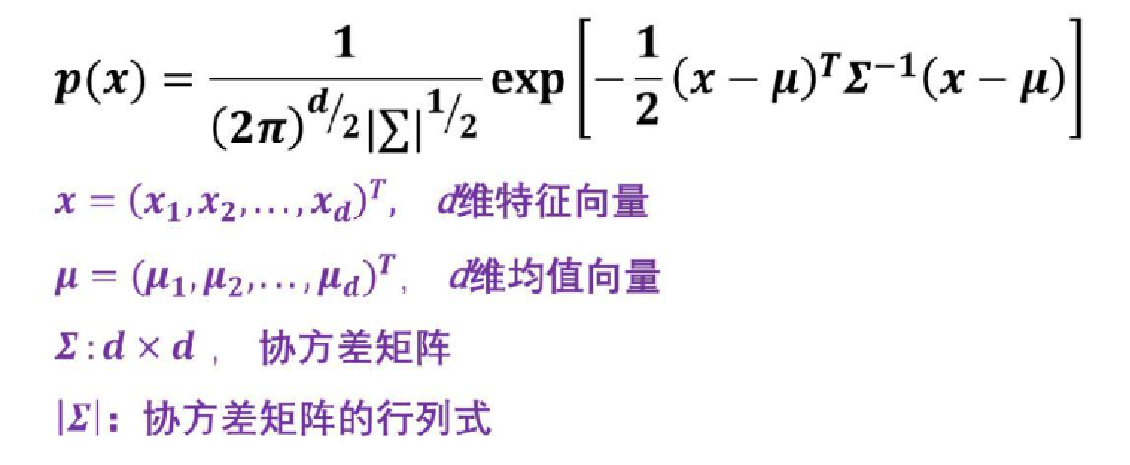因此，我们假设类条件概率符合多维正态分布，则判别函数可以写成：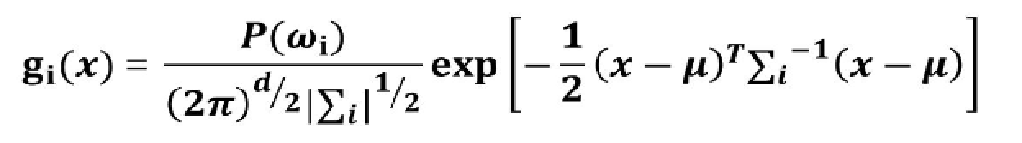由于该判别函数含有指数，不方便计算。故取对数得到新的判别函数：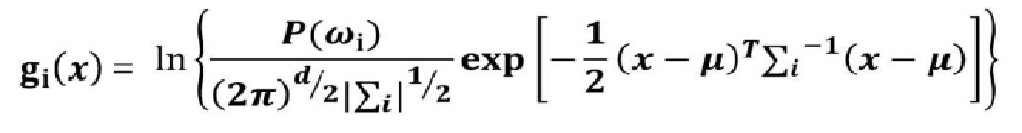将其化简为：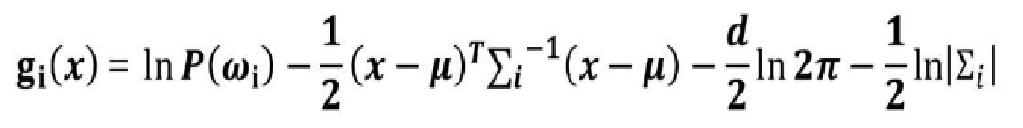由于$\frac{d}{2}\ln (2π)$与类别无关，所以判别函数可以进一步简化为：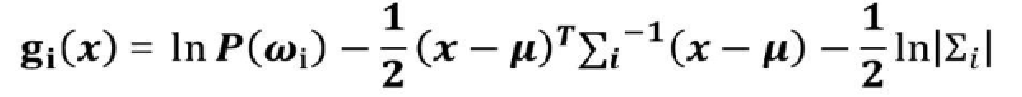我们后面的实验过程就是根据此判别函数作为样本区分的依据。
三、MATLAB仿真实现
方案一：利用MATLAB自带的贝叶斯分类工具
clear
clc
load wine.data
wine_class = wine;

%训练集与测试集的划分
data_train = [wine_class(1:35,:);wine_class(55:115,:);wine_class(131:163,:)];
data_test = [wine_class(36:54,:);wine_class(116:130,:);wine_class(164:178,:)];

train_num = length(data_train);
test_num = length(data_test);
train_labels1 = data_train(:,1);
test_labels1 = data_test(:,1);
% 这里对数据进行一次处理。贝叶斯分类器要求输入数据中同类别中一个特征的方差不能为0
% 由公式我们可以知道，计算后验概率时，如果某一列特征全部相同，那么后验概率计算时分母会变为0，
% 为了避免该种情况，需要对数据进行提前处理

[data_train,position] = data_proc(data_train,train_labels1(1:train_num)');
%  对训练数据进行处理后，同时也要对测试数据进行同样的处理
for rows = 1:10
data_test(:,position{1,rows})=[];
end

%模型部分
% 超参数全部取了默认值，比较重要的，如类别的先验概率，如果不进行修改，则计算输入数据中类别的频率
% 查看nb_model即可确认所使用的超参数
nb_model = fitcnb(data_train,train_labels1(1:train_num));
%训练模型
%测试结果
result = predict(nb_model,data_test);
result = result.';
fprintf('预测结果：');
result(1:test_num)
%取30个打印出来对比
fprintf('真实分布：');
test_labels1(1:test_num)'
% 整体正确率
acc = 0.;
for i = 1:test_num
if result(i)==test_labels1(i)
acc = acc+1;
end
end
fprintf('精确度为：%5.2f%%\n',(acc/test_num)*100);

% data_proc.m
% 函数功能：删除同类数据特征中方差为0的特征列
% 输入：行向量数据及标签
% 输出：删除列之后的数据以及删除的列标
function [output,position] = data_proc(data,label)
position = cell(1,10);
%创建cell存储每类中删除的列标
for i = 0:9
temp = [];
pos = [];
for rows = 1:size(data,1)
if label(rows)==i
temp = [temp;data(rows,:)];
end
end
for cols = 1:size(temp,2)
var_data = var(temp(:,cols));
if var_data==0
pos = [pos,cols];
end
end
position{i+1} = pos;
data(:,pos)=[];
end
output = data;
end


分类精确度是一个固定值，为：97.96%说明：（1）此代码采用的MATLAB自带的函数工具，分别为训练模型函数fitcnb()与预测模型函数predict()。两个函数的用法可以参考MATLAB软件：【帮助】，里面有相关函数的解释。（2）此代码中的训练集与测试集的划分完全是根据自己的设置调整的，不是十分地严谨。（3）代码中没有对数据进行归一化处理。在实际中，数据集中每个属性对应的值可能相差很大，此时就需要归一化处理，将不同类型的特征数值大小变为一致，方便数据处理。

方案二：自编函数实现贝叶斯分类算法
clear
clc
load wine.data
wine_class = wine;
% mapminmax为MATLAB自带的归一化函数
[wine_class,ps] = mapminmax(wine_class(:,2:size(wine_class,2))',0,1);      %归一化要先转至
wine_class = [wine(:,1),wine_class'];

% 训练集和测试集的划分
% K-折交叉验证(k-fold crossValidation)
[wine_class_r, wine_class_c] = size(wine_class);
K=10;
sum_accuracy = 0;
%将数据样本随机分割为10部分
indices = crossvalind('Kfold', wine_class_r,K);
for i = 1 :K
% 获取第i份测试数据的索引逻辑值
test = (indices == i);
% 取反，获取第i份训练数据的索引逻辑值
train = ~test;
%1份测试，9份训练
test_data = wine_class(test, 2 : wine_class_c);
test_label = wine_class(test, 1);
train_data = wine_class(train, 2 : wine_class_c );
train_label = wine_class(train, 1);
%模型的训练以及预测结果
train_model = Naive_Bayesian( train_data,train_label);
result = predict_BN(train_model,test_data,test_label);
accuracy_test = sum(result == test_label) / length(test_label)*100;
sum_accuracy = sum_accuracy + accuracy_test;
end
%求平均准确率
mean_accuracy = sum_accuracy / K;
disp('平均准确率：');
fprintf('%15.2f%%\n',mean_accuracy)

function [model]= Naive_Bayesian(X, Y)
% 朴素贝叶斯分类器的实现
m=size(X,1);%记录训练集样本总数
n=size(X,2);%记录训练集特征属性数目
%记录类别数及每个类别编号
yy=Y(:);
yy=sort(yy);
d=diff([yy;max(yy)+1]);%差分计算
count = diff(find([1;d]));%记录每个类别出现次数//find返回非零元素的索引位置
class_num=yy(find(d));%记录类别编号[1;2;3]
%计算每个类别的先验概率 p(W)
for i=1:length(class_num)
pW(i) = count(i)/m;
end
x1 = X(1:count(1),:); %第一类样本
x2 = X(count(1)+1:count(1)+count(2),:);   %第二类样本
x3 = X(count(1)+count(2)+1:count(1)+count(2)+count(3),:);   %第三类样本
x = {x1;x2;x3};
for i = 1:length(class_num)
smean{i} = mean(cell2mat(x(i))); %求各类别的均值
scov{i} = cov(cell2mat(x(i)));  %求各类别的协方差矩阵
end
for i = 1:length(class_num)
sinv{i} = pinv(cell2mat(scov(i))); %求各类别协方差矩阵的逆矩阵
sdet{i} = det(cell2mat(scov(i)));  %求各类别协方差矩阵的行列式
end
model.ssmean = smean;
model.sscov = scov;
model.ssinv = sinv;
model.ssdet = sdet;
model.pW = pW;
end

function result = predict_BN(model,X,Y)
%朴素贝叶斯分类器的预测
ssmean = model.ssmean ;
sscov = model.sscov ;
ssinv = model.ssinv ;
ssdet = model.ssdet ;
pW = model.pW;
m = size(X,1);%记录测试样本总数
n = size(X,2);%记录测试样本特征数13
%记录每个类别编号
yy=Y(:);
yy=sort(yy);
d=diff([yy;max(yy)+1]);%差分计算
class_num=yy(find(d));%记录类别编号
%求判别函数gi(x)
for i = 1:length(class_num)
for j = 1:length(X)
gi(j,i) =log(pW(i))-(1/2)*(X(j,:)'-cell2mat(ssmean(i))')'*(cell2mat(ssinv(i)))*(X(j,:)'-cell2mat(ssmean(i))')-(1/2)*log(cell2mat(ssdet(i)));
end
end
for i=1:m
[max_Pro(i,1), index] = max(gi(i,:));%输出处于最大概率的概率值以及其对应的索引位置
result(i,1)=class_num(index);%待测样本的预测类别
end
end


每次运行的分类精确度不同：大致在98%~100%说明：（1）代码中数据的归一化函数采用的是mapminmax()。（2）训练集与测试集划分不是随意划分的，也不是根据准确率的高低来调整训练集与测试集，而是采用k折交叉验证（K=10），k-折交叉验证的相关知识请大家咨询一下其他博客，本文不一一赘述。（3）后面朴素贝叶斯分类训练模型Naive_Bayesian()与预测模型predict_BN()是自己根据上面讲述的判别函数自编的。（4）在10折交叉验证中，每次运行都有10次分类结果，将10次分类结果取平均值，就可以得到比较准确的分类精度。

写到这里很不易，感谢大家大家滴观看！有错误敬请指正，看到尽量根据水平改。


展开全文• 基于最小风险贝叶斯决策的分类器，模式识别实验报告，word版，里面有实验原理、matlab源程序及运行结果和输出图像。
• bayes是对细胞的判别分类 分为最小错误率和最小风险两种 fisher是对两类进行判别
• matlab 编写的bayes、fisher分类器，适合初学者，程序说明也比较清楚
• 基于MOOC人工智能之模式识别的课程完成的第二次作业 MATLAB实现贝叶斯算法（MNIST数据集） MOOC地址：人工智能之模式识别 ...因此，只要知道先验概率 P(ωj)，类条件概率密度 p(x/ωj) 就可以设计出一个贝叶斯分类器
基于MOOC人工智能之模式识别的课程完成的第四次作业
MATLAB实现贝叶斯算法（MNIST数据集）
MOOC地址：人工智能之模式识别
贝叶斯方法是统计模式识别理论中最基础的一种方法，它通过样本的先验概率和条件概率计算后验概率，以此来判断在条件
生时，某结果发生的概率大小。
贝叶斯分类器的基础是贝叶斯公式。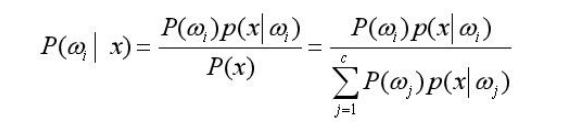它的分类决策规则是：依据计算得到的后验概率对样本进行归类，而需要的条件是：先验概率和类条件概率已知。
因此，只要知道先验概率 P(ωj)，类条件概率密度 p(x/ωj) 就可以设计出一个贝叶斯分类器。
而 P(ωj)、p(x/ωj)并不能预先知道，需要利用训练样本集的信息去进行估计。所以，贝叶斯分类器的训练，就是从样本集中估计出先验概率和类条件概率。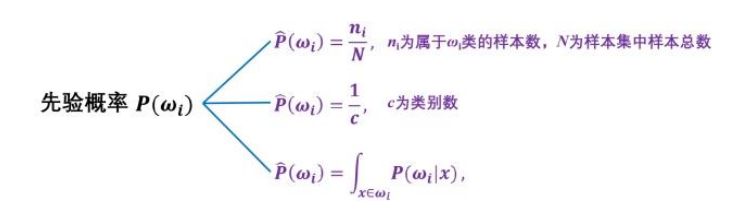Matlab 已经有贝叶斯模型相关函数，我们只需输入训练样本和相应的标签数据就可以训练得到分类器。
data_proc.m 是对图像数据的预处理函数，主要是为了解决训练样本特征空间中有的列向量全部为零，这样在计算后验概率时会出现分母为零的情况。
% data_proc.m
% 函数功能：删除同类数据特征中方差为0的特征列
% 输入：行向量数据及标签
% 输出：删除列之后的数据以及删除的列标
function [output,position] = data_proc(data,label)
position = cell(1,10);
%创建cell存储每类中删除的列标
for i = 0:9
temp = [];
pos = [];
for rows = 1:size(data,1)
if label(rows)==i
temp = [temp;data(rows,:)];
end
end
for cols = 1:size(temp,2)
var_data = var(temp(:,cols));
if var_data==0
pos = [pos,cols];
end
end
position{i+1} = pos;
data(:,pos)=[];
end
output = data;
end


Bayesian.m 程序代码主要实现了数据集读入，贝叶斯分类器训练，测试及结果输出几个部分。
%Bayesian.m
clear
clc
load ('../test_images.mat');
load ('../test_labels.mat');
load ('../train_images.mat');
load ('../train_labels.mat');
train_num = 2000;
test_num = 200;
%训练数据
data_train = mat2vector(train_images(:,:,1:train_num),train_num);
%图像转向量
data_test = mat2vector(test_images(:,:,1:test_num),test_num);
% 这里对数据进行一次处理。贝叶斯分类器要求输入数据中同类别中一个特征的方差不能为0
% 由公式我们可以知道，计算后验概率时，如果某一列特征全部相同，那么后验概率计算时分母会变为0，
% 为了避免该种情况，需要对数据进行提前处理
[data_train,position] = data_proc(data_train,train_labels1(1:train_num)');
%  对训练数据进行处理后，同时也要对测试数据进行同样的处理
for rows = 1:10
data_test(:,position{1,rows})=[];
end
%模型部分
% 超参数全部取了默认值，比较重要的，如类别的先验概率，如果不进行修改，则计算输入数据中类别的频率
% 查看nb_model即可确认所使用的超参数
nb_model = fitcnb(data_train,train_labels1(1:train_num));
%训练模型
%测试结果
result = predict(nb_model,data_test);
result = result.';
fprintf('预测结果：');
result(1:20)
%取20个打印出来对比
fprintf('真实分布：');
test_labels1(1:20)
% 整体正确率
acc = 0.;
for i = 1:test_num
if result(i)==test_labels1(i)
acc = acc+1;
end
end
fprintf('精确度为：%5.2f%%\n',(acc/test_num)*100);

通过改变训练样本的数量，分类器识别精度也会随之改变，但他们的关系并不是单调的。展开全文机器学习
• 其观察值相应的类条件概率密度如下，试利用最小错误率贝叶斯决策规则和最小风险贝叶斯决策规则，分别设计一个分类器，对它们进行分类（分为两类，正常和异常）， (结果表述格式自己设计，表达清楚每个样本的分类...
• 设计了一种基于matlab的手写数字识别系统，全面覆盖多种分类器，有Fisher线性判别，贝叶斯分类器，神经网络，k近邻等等线性与非线性的分类器，识别的准确率较高，具体依据各个算法的不同，可以在此基础上进行改进。
• 设计Bayes决策理论的随机模式分类器，用matlab实现。 Bayes分类器的基本思想是依据类的概率、概密，按照某种准则使分类结果从统计上讲是最佳的。换言之，根据类的概率、概密将模式空间划分成若干个子空间，在此基础...Bayes
•机器学习
• 宝贝，含泪分享，上述代码主要包括了线性分类器设计，贝叶斯分类器设计，动态聚类。 还有最优化的代码，包括拟牛顿法，共轭梯度法，黄金分割等等， share with you!
• 分类器设计时可以考察采用不同先验概率（如0.5对0.5, 0.75对0.25, 0.9对0.1等）进行实验，考察对决策规则和错误率的影响。 2）应用两个特征进行实验：同时采用身高和体重数据作为特征，分别假设二者相关或不相关，...
• 全书共分为13章，内容包括：模式识别概述，特征的选择与提取，模式相似性测度，贝叶斯分类器设计，判别函数分类器设计，神经网络分类器设计（BP神经网络、径向基函数冲经网络、自组织竞争神经网络、慨率神经网络、对...
• 模式识别4个实验（matlab代码 ）1.贝叶斯决策分类器对鸢尾花 2.基于Fisher 准则的线性分类器分析 3.PCA 人脸特征提取与重构 4.设计 C-均值聚类 分器对鸢尾花均值聚类
• 2、试验直接设计线性分类器的方法，与基于概率密度估计的贝叶斯分类器进行比较。 3、体会留一法估计错误率的方法和结果。 二、具体做法 1、在第一次实验中，挑选一次用身高作为特征，并且先验概率分别为男生0.5，...
用身高和体重数据进行性别分类的实验报告（二）
一、 基本要求
1、试验非参数估计，体会与参数估计在适用情况、估计结果方面的异同。
2、试验直接设计线性分类器的方法，与基于概率密度估计的贝叶斯分类器进行比较。
3、体会留一法估计错误率的方法和结果。
二、具体做法
1、在第一次实验中，挑选一次用身高作为特征，并且先验概率分别为男生0.5，女生0.5的情况。改用Parzen窗法或者kn近邻法估计概率密度函数，得出贝叶斯分类器，对测试样本进行测试，比较与参数估计基础上得到的分类器和分类性能的差别。
2、同时采用身高和体重数据作为特征，用Fisher线性判别方法求分类器，将该分类器应用到训练和测试样本，考察训练和测试错误情况。将训练样本和求得的决策边界画到图上，同时把以往用Bayes方法求得的分类器也画到图上，比较结果的异同。
3、选择上述或以前实验的任意一种方法，用留一法在训练集上估计错误率，与在测试集上得到的错误率进行比较。
三、原理简述及程序框图
1、挑选身高(身高与体重)为特征，选择先验概率为男生0.5女生0.5的一组用Parzen窗法来求概率密度函数，再用贝叶斯分类器进行分类。
以身高为例
本次实验我们组选用的是正态函数窗，即，窗宽为（h是调节的参量，N是样本个数）
，（d表示维度）。因为区域是一维的，所以体积为。Parzen公式为。
故女生的条件概率密度为
clc;
clear all;
[FH FW]=textread('C:\Users\xuyd\Desktop\homework\FEMALE.txt','%f%f');
[MH MW]=textread('C:\Users\xuyd\Desktop\homework\MALE.txt','%f%f');
FA=[FH FW];
MA=[MH MW];
N1=max(size(FA));
h1=4;
hn1=h1/(sqrt(N1));
VN1=h1/(sqrt(N1));
N2=max(size(MA));
h2=4;
hn2=h2/(sqrt(N2));
VN2=h2/(sqrt(N2));
[tH tW]=textread('C:\Users\xuyd\Desktop\homework\test2.txt','%f%f%*s');
X=[tH tW];
[M N]=size(X);
s=zeros(M,1);
A=[X(:,1) X(:,2) s];
error=0;
errorgirl=0;
errorboy=0;
errorrate=0;
errorgirlrate=0;
errorboyrate=0;
girl=0;
boy=0;
bad=0;
for k=1:M  %测试集
x=A(k);
p=0.5;%p为属于女生的先验概率，则1-p为男生的先验概率
for i=1:N1
pp(i)=1/sqrt(2*pi)*exp(-0.5*(abs(x-FA(i)))^2/(hn1^2));%pp（i）是窗函数
end
p1=sum(1/VN1*pp');
y1=1/N1*p1;%是女生的条件概率密度函数
for j=1:N2
qq(j)=1/sqrt(2*pi)*exp(-0.5*(abs(x-MA(j)))^2/(hn2^2));
end
q1=sum(1/VN2*qq');
y2=1/N2*q1;%男生的概率密度函数，即其条件概率
g=p*y1-(1-p)*y2;%g为判别函数
if g>0
if k<=50
s(k,1)=0;%判为女生
girl=girl+1;
else
errorboy=errorboy+1;
end
elseif g<0
if k<=50
errorgirl=errorgirl+1;
else
s(k,1)=1;%判为男生
boy=boy+1;
end
else
s(k,1)=-2;%不能判别是指等于0时的情况
bad=bad+1;
end
end
errorgirl
errorboy
bad
girl=errorboy+girl
boy=boy+errorgirl
error=errorgirl+errorboy
errorgirlrate=errorgirl/50
errorboyrate=errorboy/250
errorrate=error/M
%特征是身高与体重，先验概率为0.5、0.5时用Parzen窗法，贝叶斯分类器。
clc;
clear all;
[FH FW]=textread('C:\Users\xuyd\Desktop\homework\FEMALE.txt','%f%f');
[MH MW]=textread('C:\Users\xuyd\Desktop\homework\MALE.txt','%f%f');
FA=[FH FW];
MA=[MH MW];
N1=max(size(FA));
h1=7;
hn1=h1/(sqrt(N1));
VN1=hn1^2;
N2=max(size(MA));
h2=7;
hn2=h2/(sqrt(N2));
VN2=hn2^2;
[tH tW]=textread('C:\Users\xuyd\Desktop\homework\test2.txt','%f%f%*s');
X=[tH tW];
[M N]=size(X);
s=zeros(M,1);
error=0;
errorgirl=0;
errorboy=0;
errorrate=0;
errorgirlrate=0;
errorboyrate=0;
girl=0;
boy=0;
bad=0;
for k=1:M
A=[X(k,1) X(k,2)];
x=A;
p=0.5;%p为属于女生的先验概率，则1-p为男生的先验概率
pp=0;
for i=1:N1
fa=[FA(i,1) FA(i,2)];
n=1/sqrt(2*pi)*exp(-0.5*abs((x-fa)*(x-fa)')/(hn1^2));
pp=pp+n;
end
p1=1/VN1*pp';
y1=1/N1*p1;%是女生的条件概率密度函数
qq=0;
for j=1:N2
ma=[MA(j,1) MA(j,2)];
m=1/sqrt(2*pi)*exp(-0.5*abs((x-ma)*(x-ma)')/(hn2^2));
qq=m+qq;
end
q1=sum(1/VN2*qq');
y2=1/N2*q1;%男生的概率密度函数，即其条件概率
g=p*y1-(1-p)*y2;%g为判别函数
if g>0
if k<=50
s(k,1)=0;%判为女生
girl=girl+1;
else
errorboy=errorboy+1;
end
elseif g<0
if k<=50
errorgirl=errorgirl+1;
else
s(k,1)=1;%判为男生
boy=boy+1;
end
else
s(k,1)=-2;%不能判别是指等于0时的情况
bad=bad+1;
end
end
errorgirl
errorboy
bad
girl=errorboy+girl
boy=boy+errorgirl
error=errorgirl+errorboy
errorgirlrate=errorgirl/50
errorboyrate=errorboy/250
errorrate=error/M

%用fisher线性判别法求阈值
function [w,y0]=fisher(AA,BB)
A=AA';
B=BB';
[k1,l1]=size(A);
[k2,l2]=size(B);
M1=sum(AA);
M1=M1';
M1=M1/l1;%男生均值向量
M2=sum(BB);
M2=M2';
M2=M2/l2;%女生均值向量
S1=zeros(k1,k1);%建立矩阵
S2=zeros(k2,k2);
for i=1:l1
S1=S1+(A(:,i)-M1)*((A(:,i)-M1).');%男生的类内离散度矩阵
end
for i=1:l2
S2=S2+(B(:,i)-M2)*((B(:,i)-M2).');%女生的类内离散度矩阵
end
Sw=0.5*S1+0.5*S2;%总类内离散度矩阵,先验概率0.5
w=inv(Sw)*(M1-M2);%两列
wT=w';%wT就是使Fisher准则函数JF（w）取极大值时的解，也就是d维X空间到1维Y空间的最好的投影方向
for i=1:l1
Y1(i)=wT(1,1)*A(1,i)+wT(1,2)*A(2,i);%求出二维男生样本集映射到一维时的量
end
for i=1:l2
Y2(i)=wT(1,1)*B(1,i)+wT(1,2)*B(2,i);%求出二维女生样本集映射到一维时的量
end
m1=sum(Y1)/l1;
m2=sum(Y2)/l2;
y0=(l1*m1+l2*m2)/(l1+l2);%
%用fisher线性判别函数来判断
clc
clear all
[filename,pathname,filterindex] = uigetfile('*.txt', '请读入男生训练集');
fileAddrs = [pathname,filename];
[A1 A2]=textread(fileAddrs,'%f%f');
[filename,pathname,filterindex] = uigetfile('*.txt', '请读入女生训练集');
fileAddrs = [pathname,filename];
[B1 B2]=textread(fileAddrs,'%f%f');
AA=[A1 A2];
BB=[B1 B2];
[w,y0]=fisher(AA,BB);
wT=w';
girl=0;
boy=0;
bad=0;
errorgirl=0;
errorboy=0;
error=0;
errorgirlrate=0;
errorboyrate=0;
errorrate=0;
[filename,pathname,filterindex] = uigetfile('*.txt', '请读入测试集');
fileAddrs = [pathname,filename];
[T1 T2]=textread(fileAddrs,'%f%f%*s');
TT=[T1 T2];T=TT';
[k3 l3]=size(T);
for k=1:50
y(k)=wT*T(:,k);
if y(k)>y0
errorgirl=errorgirl+1;
else if y(k)<y0
girl=girl+1;
else
bad=bad+1;
end
end
end
for k=51:300
y(k)=wT*T(:,k);
if y(k)>y0
boy=boy+1;
else if y(k)<y0
errorboy=errorboy+1;
else
bad=bad+1;
end
end
end
errorgirl
errorboy
bad
girl=errorboy+girl
boy=boy+errorgirl
error=errorgirl+errorboy
errorgirlrate=errorgirl/50
errorboyrate=errorboy/250
errorrate=error/l3
%画图

[filename,pathname,filterindex] = uigetfile('*.txt', '请读入男生训练集');
fileAddrs = [pathname,filename];
[A1 A2]=textread(fileAddrs,'%f%f');
[filename,pathname,filterindex] = uigetfile('*.txt', '请读入女生训练集');
fileAddrs = [pathname,filename];
[B1 B2]=textread(fileAddrs,'%f%f');
AA=[A1 A2];
BB=[B1 B2];
A=AA';
B=BB';
[k1,l1]=size(A);
[k2,l2]=size(B);
[w,y0]=fisher(AA,BB);
for i=1:l1
x=A(1,i);
y=A(2,i);%x是身高，y是体重
plot(x,y,'R.');
hold on
end
for i=1:l2
x=B(1,i);
y=B(2,i);
plot(x,y,'G.');
hold on
end
a1=min(A(1,:));%男生身高最小值
a2=max(A(1,:));%男生身高最大值
b1=min(B(1,:));%女生身高最小值
b2=max(B(1,:));%女生身高最大值
a3=min(A(2,:));%男生体重最小值
a4=max(A(2,:));%男生体重最大值
b3=min(B(2,:));%女生体重最小值
b4=max(B(2,:));%女生体重最大值
if a1<b1
a=a1;
else
a=b1;%a是所有人中身高最小值
end
if a2>b2
b=a2;
else
b=b2;%b是所有人中身高最大值
end
if a3<b3
c=a3;
else
c=b3;%c是所有人中体重最小值
end
if a4>b4
d=a4;
else
d=b4;%d为所有人中体重最大值
end
x=a:0.01:b;
y=(y0-x*w(1,1))/w(2,1);
plot(x,y,'B');
hold on;
%身高体重相关,判别测试样本
%手动先验概率
P1=0.5;
P2=0.5;
FA=A;
MA=B;
a=cov(FA')*(length(FA)-1)/length(FA);
b=cov(MA')*(length(MA)-1)/length(MA);
W1=-1/2*inv(a);
W2=-1/2*inv(b);
Ave1=(sum(FA')/length(FA))';
Ave2=(sum(MA')/length(MA))';
w1=inv(a)*Ave1;
w2=inv(b)*Ave2;
w10=-1/2*Ave1'*inv(a)*Ave1-1/2*log(det(a))+log(P1);
w20=-1/2*Ave2'*inv(b)*Ave2-1/2*log(det(b))+log(P2);
syms x ;
syms y ;
h=[x y]';
h1=h'*W1*h+w1'*h+w10;
h2=h'*W2*h+w2'*h+w20 ;
h=h1-h2;
ezplot(h,[130,200,30,100])
%功能：应用Fisher准则判断一个身高体重二维数据的性别
vector=[x;y];
yy=(w.')*vector;
if yy>y0
value=2;%表示样本是男生
else
value=1;%表示样本是女生
end
%功能：使用留一法求训练样本错误率
[A1 A2]=textread('C:\Users\Administrator\Desktop\模式识别\homework\MALE.txt','%f%f');
[B1 B2]=textread('C:\Users\Administrator\Desktop\模式识别\homework\FEMALE.txt','%f%f');
AA=[A1 A2];
BB=[B1 B2];
A=AA';
B=BB';
m1=2;
m2=2;
n1=50;
n2=50;
tempA=zeros(m1,n1-1);
count=0;
for i=1:n1
for j=1:(i-1)
tempA(:,j)=A(:,j);
end
for j=(i+1):n1
tempA(:,j-1)=A(:,j);
end
[w,y0]=fisher((tempA.'),BB);
flag=classify_CH(A(1,i),A(2,i),w,y0);
if flag==1
count=count+1;
end
end
tempB=zeros(m2,n2-1);
for i=1:n2
for j=1:(i-1)
tempB(:,j)=B(:,j);
end
for j=(i+1):n2
tempB(:,j-1)=B(:,j);
end
[w,y0]=fisher(AA,(tempB.'));
flag=classify_CH(B(1,i),B(2,i),w,y0);
if flag==2
count=count+1;
end
end
error_ratio=count/(n1+n2)
%使用留一法求测试样本错误率
[T1 T2]=textread('C:\Users\Administrator\Desktop\模式识别\homework\test2.txt','%f%f%*s');
TT=[T1 T2];
T=TT';
[k3 l3]=size(T);
TG=zeros(2,50);
TB=zeros(2,250);
for i=1:50
TG(:,i)=T(:,i);
end
for j=51:l3
TB(:,j-50)=T(:,j);
end
m1=2;
m2=2;
n1=50;
n2=250;
tempA=zeros(m1,n1-1);
count=0;
for i=1:n1
for j=1:(i-1)
tempA(:,j)=TG(:,j);
end
for j=(i+1):n1
tempA(:,j-1)=TG(:,j);
end
[w,y0]=fisher((tempA.'),TB');
flag=classify_CH(TG(1,i),TB(2,i),w,y0);
if flag==1
count=count+1;
end
end
tempB=zeros(m2,n2-1);
for i=1:n2
for j=1:(i-1)
tempB(:,j)=TB(:,j);
end
for j=(i+1):n2
tempB(:,j-1)=TB(:,j);
end
[w,y0]=fisher(TG',(tempB.'));
flag=classify_CH(TB(1,i),TB(2,i),w,y0);
if flag==2
count=count+1;
end
end
error_ratio=count/(n1+n2)
展开全文算法 计算机视觉
• [模式识别与智能计算：MATLAB技术...扫描版 内容包括：模式识别概述、特征选择与优化、模式相似性测度、贝叶斯分类、各神经网络的分类器设计、决策树以及禁忌搜索、遗传算法、蚁群算法等等。实践步骤是以matlab为基础深度学习
• 第1篇为MATLAB常用算法应用设计，包括贝叶斯分类器的数据处理、背景差分的运动目标检测、小波变换的图像压缩、BP的模型优化预测、RLS算法的数据预测、GA优化的BP网络算法分析、分形维数应用、碳排放约束下的煤炭消费...
• 关于贝叶斯分类器设计的实验，适合入门，用matlab实现
• 7.4.1 模式分类贝叶斯决策理论/205 7.4.2 概率神经网络的结构/206 7.4.3 概率神经网络的优点/207 7.5 广义回归神经网络/208 7.5.1 广义回归神经网络的理论基础/208 7.5.2 广义回归神经网络的结构/209 7.6 径向基...
• 讨论了贝叶斯分类、贝叶斯网络、线性和非线性分类器设计、特征生成、特征选取技术、学习理论的基本概念以及聚类概念与算法。与前一版相比，增加了大数据集和高维数据相关的*算法，提供了*的分类器和鲁棒回归的核方法...
• Stanford机器学习课程笔记2-高斯判别分析与朴素贝叶斯 Stanford机器学习课程笔记3-学习理论部分 Stanford机器学习课程笔记4-Kmeans与高斯混合模型 深度卷积网络CNN与图像语义分割 OpenCV系列 CentOS上编译安装...
• 目前Shogun的机器学习功能分为几个部分：feature表示，feature预处理，核函数表示,核函数标准化，距离表示，分类器表示，聚类方法，分布，性能评价方法，回归方法，结构化输出学习器。 SHOGUN 的核心由C++实现，...
• 数据科学 - Python / R / Julia / Matlab 机器学习 - Python / R / C++ / Julia 自动化测试 - Python / Shell 作为一名Python开发者，根据个人的喜好和职业规划，可以选择的就业领域也非常多。 Python后端开发...# matlab贝叶斯分类器设计matlab 订阅